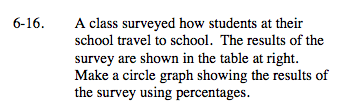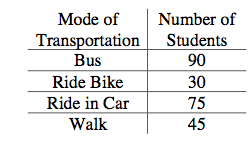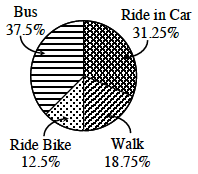### Home > MC2 > Chapter 6 > Lesson 6.1.2 > Problem6-16

6-16.Find the students using each mode of transportation as a percentage of all the students.

Each category of data is then represented by a portion of the circle. The measure of the angle of each sector portion is directly proportional to the corresponding percentage.

Consider the bike riders.

$\frac{30}{240}=0.125\: \text{of\:the\:student}$

$\text{population\: ride\: bikes\: to\: school.}$

Proportionately as an angle, this would be 12.5% of 360° (a whole circle). This equates to 0.125(360°) = 45°.